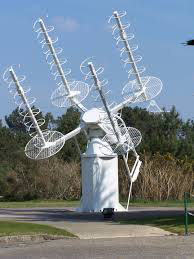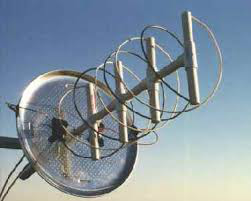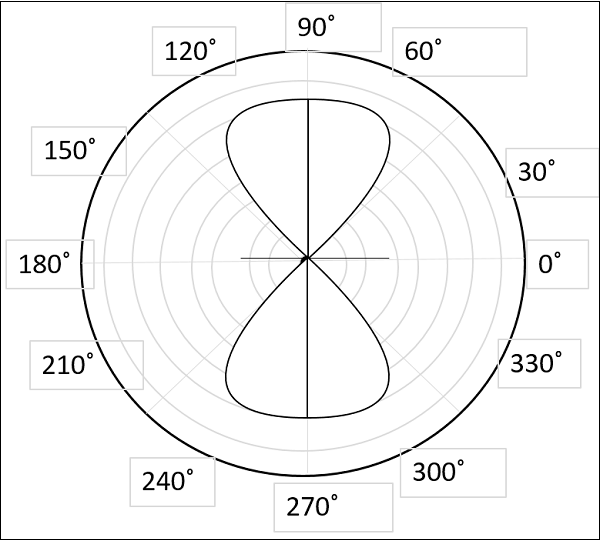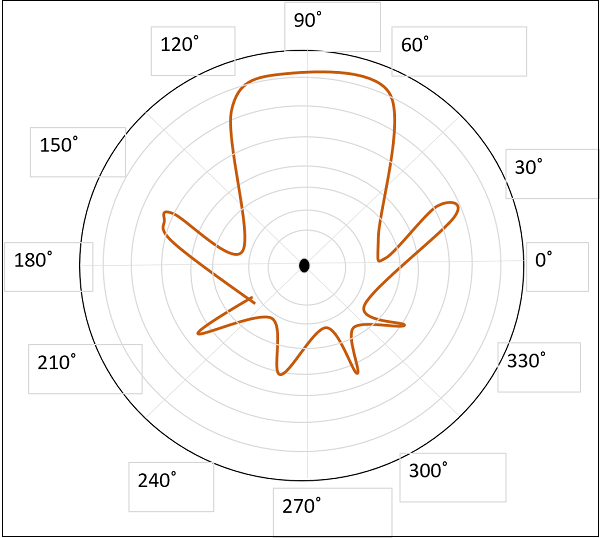# Antenna Theory - Helical

Helical antenna is an example of wire antenna and itself forms the shape of a helix. This is a broadband VHF and UHF antenna.

### Frequency Range

The frequency range of operation of helical antenna is around 30MHz to 3GHz. This antenna works in VHF and UHF ranges.

## Construction & Working of Helical AntennaHelical antenna or helix antenna is the antenna in which the conducting wire is wound in helical shape and connected to the ground plate with a feeder line. It is the simplest antenna, which provides circularly polarized waves. It is used in extra-terrestrial communications in which satellite relays etc., are involved.

The above image shows a helical antenna system, which is used for satellite communications. These antennas require wider outdoor space.

It consists of a helix of thick copper wire or tubing wound in the shape of a screw thread used as an antenna in conjunction with a flat metal plate called a ground plate. One end of the helix is connected to the center conductor of the cable and the outer conductor is connected to the ground plate.The image of a helix antenna detailing the antenna parts is shown above.

The radiation of helical antenna depends on the diameter of helix, the turn spacing and the pitch angle.

Pitch angle is the angle between a line tangent to the helix wire and plane normal to the helix axis.

$$\alpha = \tan^{-1}(\frac{S}{\pi D})$$

where,

• D is the diameter of helix.

• S is the turn spacing (centre to centre).

• α is the pitch angle.

## Modes of Operation

The predominant modes of operation of a helical antenna are −

• Normal or perpendicular mode of radiation.

• Axial or end-fire or beam mode of radiation.

Let us discuss them in detail.

### Normal mode

In normal mode of radiation, the radiation field is normal to the helix axis. The radiated waves are circularly polarized. This mode of radiation is obtained if the dimensions of helix are small compared to the wavelength. The radiation pattern of this helical antenna is a combination of short dipole and loop antenna.The above figure shows the radiation pattern for normal mode of radiation in helical antenna.

It depends upon the values of diameter of helix, D and its turn spacing, S. Drawbacks of this mode of operation are low radiation efficiency and narrow bandwidth. Hence, it is hardly used.

### Axial mode

In axial mode of radiation, the radiation is in the end-fire direction along the helical axis and the waves are circularly or nearly circularly polarized. This mode of operation is obtained by raising the circumference to the order of one wavelength (λ) and spacing of approximately λ/4. The radiation pattern is broad and directional along the axial beam producing minor lobes at oblique angles.The figure shows the radiation pattern for axial mode of radiation in helical antenna.

If this antenna is designed for right-handed circularly polarized waves, then it will not receive left-handed circularly polarized waves and vice versa. This mode of operation is generated with great ease and is more practically used.

The following are the advantages of Helical antenna −

• Simple design
• Highest directivity
• Wider bandwidth
• Can achieve circular polarization
• Can be used at HF & VHF bands also

The following are the disadvantages of Helical antenna −

• Antenna is larger and requires more space
• Efficiency decreases with number of turns

### Applications

The following are the applications of Helical antenna −

• A single helical antenna or its array is used to transmit and receive VHF signals

• Frequently used for satellite and space probe communications

• Used for telemetry links with ballastic missiles and satellites at Earth stations

• Used to establish communications between the moon and the Earth